Definition Of Mixed Fraction

A Mixed Fraction is a number with a combination of an integer and a proper fraction.

Mixed fraction is also called as mixed number.
An improper fraction can be converted into a mixed fraction and vice versa.

Examples of Mixed Fraction

23, 46, 19, 5, 12, 29, 8, 42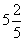is a mixed number, in which 5 is an integer and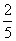is a fraction.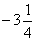is a mixed number, in which - 3 is an integer and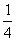is a fraction.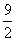is an improper fraction as the numerator is greater than the denominator.
It can be converted into a mixed fraction as: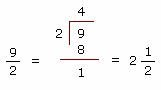So, the mixed numberis equivalent to improper fraction.

Solved Example on Mixed Fraction

Ques: Which of the following is a mixed fraction?

Choices:

A. 3 +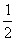B.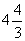C.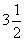D.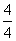Step 2: So,is a mixed fraction.